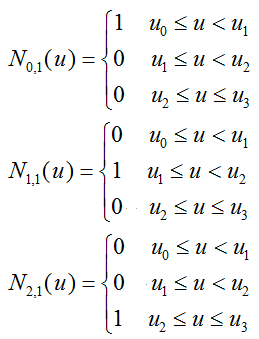﻿ B-样条曲线

## B-样条曲线

B样条曲线的定义

节点向量（u0，u1，...，un+k）所包含的n+k个区间并非都在该曲线的定义域内，其中两端各k-1个节点区间，不能作为B样条曲线的定义区间。这是因为n+1个顶点中最前的k个顶点pi，(i=0，1，...，k-1)定义了样条曲线的首段曲线，其定义区间为u∈[uk-1，uk)；随后的k个顶点Pi，(i=1，2，...，k)定义了第二段曲线，其定义区间为u∈[uk，uk+1)；...；最后的k个顶点Pi，(i=n-k+1，n-k+2，...，n)定义了末段曲线，其定义区间为u∈[un，un+1]。于是，得到k阶B样条曲线的定义域为u∈[uk-1，un+1]。共含有n-k+2个节点区间(包括零长度的节点区间)。若其中不含重节点，则对应B样条曲线包含n-k+2段。也可看到，节点向量两侧各k-1个节点区间上的那些B样条基函数因其权性不成立，不能构成基函数。

1）对n+1个控制点，曲线由n+1个混合函数所描述。
2）每个混合函数Ni,k(u)定义在u取值范围的k个子区间，以节点向量值ui为起点。
3）参数u的取值范围由n+k+1个给定节点向量值分成n+k个子区间。
4）节点向量(u0，u1，...，un+k)所生成的B样条曲线仅定义在从节点值uk-1到节点值un+1的区间上。
5）任一控制点可以影响最多k个曲线段的形状。
6）P(u)是分段参数多项式，P(u)在每一区间u∈[ui，ui+1]，(k-1≤i≤n)上都是次数不高于k-1的多项式。

从B样条曲线的这个递归定义可以看出，曲线与给定的阶数k及节点向量都有关系。就是说，即使k相同，选择不同的节点向量，也能得到不同的曲线。

任意的一阶B样条曲线就是控制点本身，可以看作是零次多项式。例如，n=2,k=1，控制点是P0，P1，P2，这样应选择参数节点n+k+1=4个，设节点向量是(u0，u1，u2，u3)，按照定义，可写出三个基函数：P0，P1，P2，P3为控制点

[0, 2]、[1, 3]为两条二次样条曲线的支撑区间。(0, 1, 2, 3)为节点向量。

```using System;
using System.Collections.Generic;
using System.ComponentModel;
using System.Data;
using System.Drawing;
using System.Linq;
using System.Text;
using System.Windows.Forms;

namespace WindowsFormsAppB
{
public partial class Form1 : Form
{
public Form1()
{
InitializeComponent();
}

public void PaintControlPoint(PaintEventArgs e, BSpline.Point[] CP)
{
Graphics g = e.Graphics;
//绘制控制点
Pen dotPen = new Pen(Color.Red, 2);
for (int i = 0; i < CP.Length; i++) {
g.DrawEllipse(dotPen, (float)CP[i].x, (float)CP[i].y, 3, 3);
}

//绘制控制点连线
Pen linePen = new Pen(Color.Red, 1);
for (int i = 1; i < CP.Length; i++) {
g.DrawLine(linePen, (float)CP[i].x, (float)CP[i].y, (float)CP[i-1].x, (float)CP[i-1].y);
}
}

public void PaintCurvePoints(PaintEventArgs e, BSpline.Point[] pts)
{
Graphics g = e.Graphics;
Pen myPen = new Pen(Color.Blue, 2);
for (int i = 0; i < pts.Length; i++) {
g.DrawEllipse(myPen, (float)pts[i].x, (float)pts[i].y, 1, 1);
}
}

public void PaintCurveLerp(PaintEventArgs e, BSpline.Point[] CP, double[] knot, double u)
{
BSpline.Point tp = BSpline.Lerp(CP, knot, u);
Graphics g = e.Graphics;
Pen myPen = new Pen(Color.Green, 2);
g.DrawEllipse(myPen, (float)tp.x, (float)tp.y, 1, 1);
}

private void Form1_Paint(object sender, PaintEventArgs e)
{
int k = 3;

//控制点
BSpline.Point[] CP = {
//加个重复节点,让曲线经过起始控制点
new BSpline.Point{ x=0, y=100},
new BSpline.Point{ x=0, y=100},
new BSpline.Point{ x=100, y=0},
new BSpline.Point{ x=200, y=100},
new BSpline.Point{ x=300, y=0},
new BSpline.Point{ x=400, y=100},
//加个重复节点,让曲线经过终止控制点
new BSpline.Point{ x=400, y=100}
};

//节点向量
double[] knot =
{
0, 100, 200, 300, 400, 500, 600, 700, 800, 900
};

//一次性计算出所有插值点
BSpline.Point[] pts = BSpline.SplinePoints(CP, CP.Length - 1, knot, 100);
PaintCurvePoints(e, pts);

//控制点
BSpline.Point[] CP1 = {
new BSpline.Point{ x=0, y=300},
new BSpline.Point{ x=0, y=300},
new BSpline.Point{ x=100, y=200},
new BSpline.Point{ x=200, y=300},
new BSpline.Point{ x=300, y=200},
new BSpline.Point{ x=400, y=300},
new BSpline.Point{ x=400, y=300}
};
//------end

//用插值方式绘制曲线
double u0 = knot[k - 1];//起始插值点
for (double u=u0; u<=700; u+=5)
{
PaintCurveLerp(e, CP1, knot, u);
}
//------end

//绘制控制点
PaintControlPoint(e, CP);
PaintControlPoint(e, CP1);
}
}

/// <summary>
/// B-样条曲线
/// </summary>
public class BSpline
{
private const int k = 3; //三次B-样条曲线

/// <summary>
/// 插值方法
/// </summary>
/// <param name="CP">控制点坐标</param>
/// <param name="knot">曲线结点向量</param>
/// <param name="u">插值点</param>
/// <returns></returns>
public static Point Lerp(Point[] CP, double[] knot, double u)
{
int n = CP.Length;
int i = 0;
while ((i < n) && (u > knot[i + 1])) i++;
return Deboor(CP, i, k, knot, u);
}

/// <summary>
/// 计算曲线离散点序列
/// </summary>
/// <param name="CP">控制点坐标</param>
/// <param name="n">控制点个数</param>
/// <param name="k">曲线的阶数</param>
/// <param name="knot">曲线结点向量</param>
/// <param name="npoints">要计算出的离散点个数</param>
/// <returns>采用德布尔(de Boor)算法生成的曲线上的离散点序列pts</returns>
public static Point[] SplinePoints(Point[] CP, int n, double[] knot, int npoints)
{
Point[] pts = new Point[npoints];

double u, delt;
int i, j;
//在每个节点区间，将参数t变化区间进行npoints等分
delt = (knot[n + 1] - knot[k - 1]) / (double)npoints;
i = k - 1;
u = knot[k-1];
for(j=0; j<npoints; j++)
{
//确定参数u所在的节点区间[ui, ui+1)
while ((i < n) && (u > knot[i + 1])) i++;
//在每个节点区间，分别求出npoints个离散点pts的坐标
pts[j] = Deboor(CP, i, k, knot, u);
u += delt;
}
return pts;
}

/// <summary>
/// 用德布尔(de Boor)算法计算出插值点u的坐标
/// 结点数(m)=控制点数(n)+次数(p)+1
/// 举例: 14个控制点定义的6次B-样条曲线，其节点的数目是21=14+6+1
/// </summary>
/// <param name="CP">控制点坐标</param>
/// <param name="i">第i个曲线段, i∈[0, n+k-l]</param>
/// <param name="k">曲线的阶数</param>
/// <param name="knot">曲线结点向量</param>
/// <param name="u">变化范围为[ui, ui+1)</param>
/// <returns>曲线在参数为t的坐标值</returns>
public static Point Deboor(Point[] CP, int i, int k, double[] knot, double u)
{
double denom, alpha;
Point[] p = new Point[k];
const double epsilon = 0.0005;
int index = 0;
//p[]存放要参与计算的控制点
for (int l = 0; l < k; l++)
{
index = i - k + l + 1;
if (index < 0)
p[l] = CP.Clone();
else if (index >= CP.Length)
p[l] = CP[CP.Length - 1].Clone();
else
p[l] = CP[index].Clone();
}

//进行k-1次循环，即进行k-1级递推
for(int r=1; r<k; r++)
{
//在每一级递推中，按照递减的顺序对控制顶点进行更新
//按递减顺序更新，是为了确保已更新的控制顶点
//不会对未更新的控制顶点的计算产生影响
for(int m=k-1; m>=r; m--)
{
int j = m + i - k + 1;
denom = knot[j + k - r] - knot[j];//分母为前一阶曲线的支撑区间
if (Math.Abs(denom) < epsilon)
alpha = 0;
else
alpha = (u - knot[j]) / denom;
//(1 - 比例因子) * 控制点坐标 + 比例因子 * 控制点坐标
p[m].x = (1 - alpha) * p[m - 1].x + alpha * p[m].x;
p[m].y = (1 - alpha) * p[m - 1].y + alpha * p[m].y;
}
}
return p[k-1];
}

#region Point
public class Point
{
public double x;
public double y;
public double z;

public Point Clone()
{
Point p = new Point();
p.x = x;
p.y = y;
p.z = z;
return p;
}
}
#endregion
}
}
```